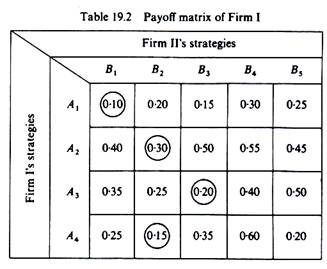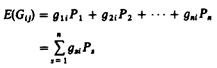# Two Person Zero-Sum Game

The simplest model is a duopoly market in which each duopolist attempts to maximise his market share.

Given this goal, whatever a firm gains (by increasing its share of the market) the other firm loses (because of the decrease in its share).

Thus any gain of one rival is offset by the loss of the other, and the net gain sums up to zero. Hence the name ‘zero-sum game’.

The assumptions of the model are:

1. The firms have a given, well-defined goal. In our particular example the goal is maximisation of the market share.
2. Each firm knows the strategies open to it and to its rival, or concentrates on the most important of these strategies.
3. Each firm knows with certainty the payoffs of all combinations of the strategies being considered. This implies that the firm knows its total revenue, total costs and total profit from each combination of strategies.
4. The actions chosen by the duopolists do not affect the total size of the market.
5. Each firm chooses its strategy ‘expecting the worst from its rival’, that is, each firm acts in the most conservative way, expecting that the rival will choose the best possible counter-strategy open to him. This behaviour is defined as ‘rational’.
6. In the zero-sum game there is no incentive for collusion, given assumption 4, since the goals of the firms are diametrically opposed.

In order to find the equilibrium solution we need information on the payoff matrix of the two firms. In our example the payoffs will be shares of the market resulting from the adoption of any two strategies by the rivals. Assume that Firm I has four strategies open to it and Firm II has five strategies. The payoff matrices of the duopolists are shown in tables 19.2 and 19.3.Clearly the sum of the payoffs in corresponding cells of the two payoff tables adds up to unity, since the numbers in these cells are shares, and the total market is shared between the two firms. In general, in the two-person zero-sum game we need not write both payoff matrices because of the nature of the game: the goals are opposing, and, in our example, the payoff table of Firm I contains indirectly information about the pay­off of Firm II. Still we start by showing both tables, and then we show how the equi­librium solution can be found from only the first payoff matrix.

Choice of strategy by Firm I:

Firm I examines the outcomes of each strategy open to it. That is, Firm I examines each row of its payoff matrix and finds the most favourable outcome of the corres­ponding strategy, because the firm expects the rival to adopt the most advantageous action open to him. This is the behavioural rule implied by assumption 5 of this model

Thus:

If Firm I adopts strategy A1, the worst outcome that it may expect is a share of 0.10 (which will be realized if the rival Firm II adopts its most favourable strategy B1).

If Firm I adopts strategy A2, the worst outcome will be a share of 0.30 (if the rival adopts the best action for him, B2).

If Firm I adopts strategy A3, the worst outcome will be a share of 0.20 (if Firm II chooses the best open alternative, B3).

If Firm I adopts strategy A4, the worst outcome will be a share of 0.15 (which would be realised by action B2 of Firm II).

Among all these minima (that is, among the above worst outcomes) Firm I chooses the maximum, the ‘best of the worst’. This is called a maximin strategy, because the firm chooses the maximum among the minima. In our example the maximin strategy of Firm I is A2, that is, the strategy which yields a share of 0.30.

Choice of strategy by Firm II:

Firm II behaves in exactly the same way. The only difference is that Firm II examines the columns of its payoff table, because these columns include the results-payoffs of each of the strategies open to Firm II. For each strategy, that is, for each column, Firm II finds the worst outcome (on the assumption that the rival will choose the best), and among these worst outcomes Firm II chooses the best. Thus, if Firm II uses its own payoff table, its behaviour is a maximin behaviour identical to the behaviour of Firm I.

However, in the zero-sum game only one payoff matrix is adequate for the equilibrium solution. In our example the first payoff table will be used not only by Firm I but also by Firm II. Thus concentrating on the first payoff table we may re­state the decision-making process of Firm II as follows. Firm II examines the columns of the (first) payoff matrix because these columns contain the information about the payoffs of its strategies.

For each column-strategy Firm II finds the maximum payoff (of Firm I) because this is the worst situation the firm (II) will face if it adopts the strategy corresponding to that column. Thus for strategy B{ the worst outcome (for Firm II) is 0-40; for strategy B2 the worst outcome is 0-30; for strategy B3 the worst outcome is 0-50; for strategy fl4 the worst result is 0-60; for strategy Bs the worst result is 0-50. Among these maxima of each column-strategy Firm II will choose the strategy with minimum value. Thus the strategy of Firm II is a minimax strategy, since it involves the choice of a minimum among the maxima payoffs. (Table 19.4.)It should be stressed that although different terms are used for the choice of the two firms (maximin behaviour of Firm I, minimax behaviour of Firm II), the behavioural rule for both firms is the same: each firm expects the worst from its rival.

In our example the equilibrium solution is strategy A2 for Firm I and B2for Firm II. This solution yields shares 0 30 for Firm I and 0-70 for Firm II. It is an equilibrium solution because it is the preferred one by both firms. This solution is called the ‘saddle point’, and the preferred strategies A2 and B2 are called ‘dominant strategies’.

It should be clear that there exists no such equilibrium (saddle) solution if there is no payoff which is preferred by both firms simultaneously. Under certain mathematical conditions other solutions and strategy choices can be determined. The analysis of the resulting mixed strategies requires a sophisticated exposition of utility theory and random selection which is beyond the scope of this book.

1. Uncertainty Model:

The assumption that each firm knows with certainty the exact value of the payoff of each strategy is unrealistic. The most probable situation in the real business world is that the firm, by adopting a certain strategy, may expect a range of results for each counter-strategy of the rival, each result with an associated probability. Thus the payoff matrix is constructed so as to include the expected value of each payoff.

The expected value is the sum of the products of the possible outcomes of a pair of strategies (adopted by the two firms) each multiplied by its probability:where gsi = the sth of the n possible outcomes of strategy i of Firm I (given that Firm II has chosen strategy j)

PS = the probability of the sth outcome of strategy i

For example, assume that Firm I chooses strategy A1 and Firm II reacts with strategy B1. This pair of simultaneous strategies may yield the shares for Firm I each with a certain probability, shown in the second column of table 19.5. Thus the expected payoff of the pair of strategies A1 and B1 is

E(G1 1) = (0.00)(0.00) + (0.05) (0.05) + (0.15)(0.05) + … + (0.95)(0.02) + (1)(0) = 0.458In a similar way we find the expected payoff of all combinations of strategies. Given the matrix of expected payoffs, the behavioural pattern of the firms is the same as in the certainty model.

That is:

Firm I adopts the maximin strategy. It finds for each row the minimum expected payoff, and among these minima the firm chooses the one with the highest value (the maximum among the minima).

Firm II adopts the minimax strategy. It finds for each column the maximum expected payoff, and among these maxima Firm II chooses the one with the smallest value (the minimum among the maxima).

Although the uncertainty zero-sum game seems simple, its assumptions are quite stringent:

1. The firms maximise their expected payoffs.
2. The zero-sum game assumes that both firms assign the same probability to each pair of payoffs; they make the same judgement. This implies that the firms must have the same information and the same objective criteria with which to evaluate the probabilities of the different payoffs. Otherwise the probability distribution of the payoffs will not be objective.
3. The firms maximise their total utility, and the utility of each payoff is proportional to the value assumed by the payoff.

The above assumptions are clearly strong and unrealistic. Furthermore, the basic condition of the zero-sum game that the ‘gain’ of one firm is equal to the ‘loss’ of the other, is rarely met in the real business world. Usually the ‘gains’ are not ‘offset’ by equal ‘losses’. Only in the case of a share goal, and in the rare case of extinction tactics, do we have a zero-sum game. In most cases we have a non-zero-sum game.

## One thought on “Two Person Zero-Sum Game”

error: Content is protected !!